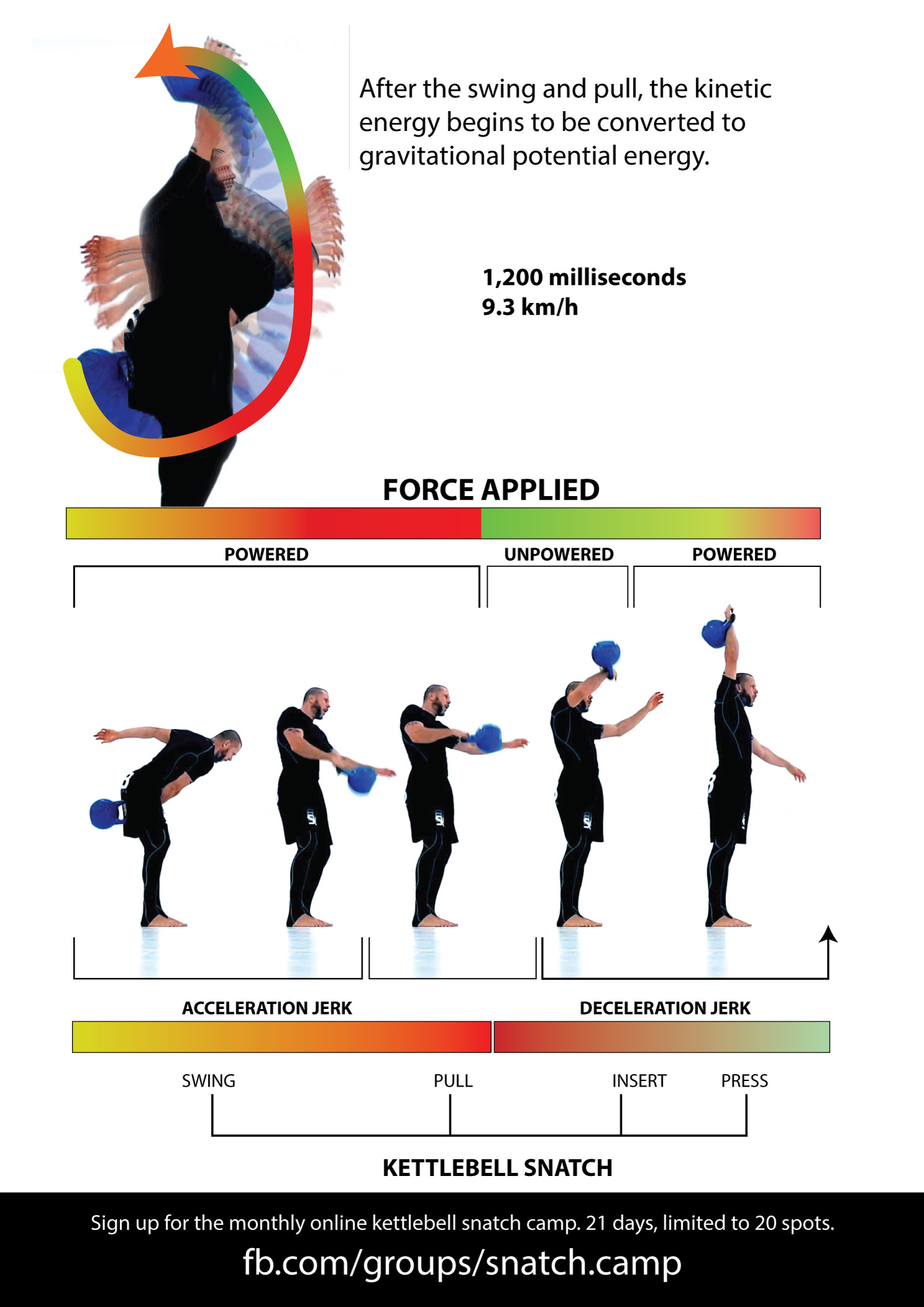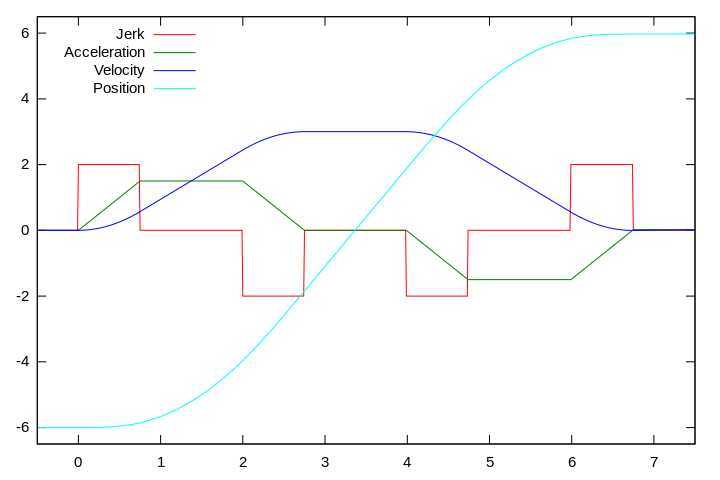# Why is the Jerk Exercise Called a Jerk? Does a Snatch Contain a Jerk?

Have you ever wondered why the Jerk exercise is called a jerk, and what exactly is a Jerk? If you have, then I have some eye-opening information for you and my theory as to why the Jerk exercise is called the Jerk.First, you need to understand that Jerk is not just a name for an exercise or a person with unlikable or obnoxious qualities and behavior, typically mean, self-centered, or disagreeable. Jerk is a term in physics that describes the rate of change of acceleration; that is, the time derivative of acceleration.

In the jerk, push press, or snatch exercise there is a jerk happening at the start and end of the exercise. With the push press there is also a press, with the (swing) snatch there is also a swing and pull, all followed by a jerk. The jerk exercise is probably the purest of them all, velocity, acceleration, and jerk, hence my theory as to why it’s been named the jerk.

Just about any exercise in which an object is accelerated will contain a jerk, not the jerk exercise, but the jerk (physics).

I could, of course, be completely wrong, but I’ve not been able to find any concrete information about the naming, if you have or know of any, please post below.1. acceleration build-uplimit jerk implies a linear increase of acceleration to the limit accelerationa quadratic increase of speed
2. limit acceleration: implies zero jerk and linear increase of speed
3. acceleration ramp-down: approaching the desired limit velocity with a negative limit jerk, i.e. linear decrease of acceleration, (negative) quadratic increase of speed
4. limit speed: implies zero jerk and zero acceleration
5. deceleration build-up: limit negative jerk implies linear decrease of acceleration to the negative limit acceleration(negative) quadratic decrease of speed
6. limit deceleration: implies zero jerk and a linear decrease of speed
7. deceleration ramp-down: limit jerk implies a linear increase of acceleration to zero, a quadratic decrease of speed, approaching the desired position at zero speed and zero acceleration
Share the Knowledge